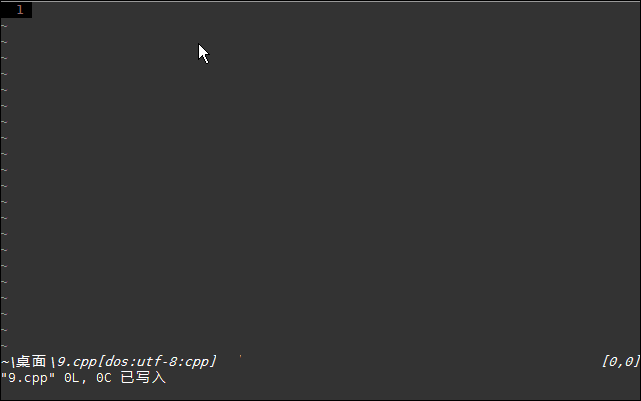# 标签归档：neocomplcache

## 从auto.vim想到的

``script karma    Rating 145/51, Downloaded by 327````````ino <= <space><=<space>
ino *= <space>*=<space>
ino /= <space>/=<space>
ino >> <space>>><space>
ino << <space><<<space>
ino >= <space>>=<space>
ino == <space>==<space>
ino += <space>+=<space>
ino ...``````